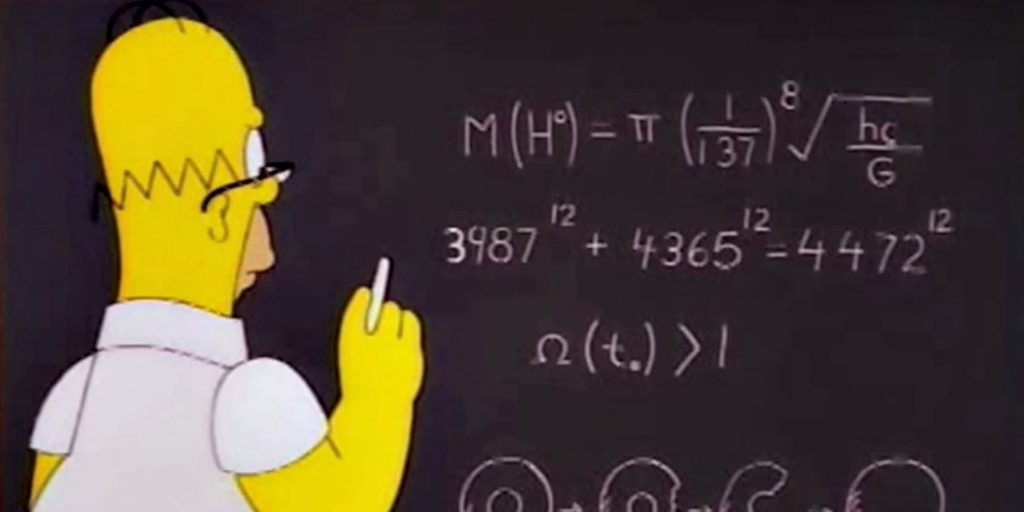# Interesting Questions On Progressions – 2IIMQuestion

Consider a set of numbers from 1 to 751. How many Arithmetic Progressions can be formed from this set of numbers such that the first term is 1 and the last term is 751 and the AP has at least 3 terms.

Solution

Numbers from 1 to 751 such that the first term is one and the last term is 751. From the basic definition of AP,

a = 1, a + (n-1)d = 751.

Subtracting both, we get -> (n-1)d = 750.

Now, if we find the number of ways 750 can be written as a product of two numbers, we have our answer. (After excluding cases that have n<3)

750 can be written as -> 1 x 750, 2 x 375, 3 x 250, 5 x 150 … and so on.

1 x 750 is a case and so is 750 x 1, this is the same with all other ways of writing the product. Effectively, if we count the number of factors in the number, then we should have the answer.

750 = 53 x 21 x 31. => Number of factors = 4 x 2 x 2 = 16.

Now we need to exclude the case where n will be lesser than 3, this means that (n-1) should be greater than or equal to 2.

One case gets eliminated -> 1 x 750.

So totally there are 16 – 1 = 15 cases.

Answer = 15

Question

Let Ai be a sequence such that An/An-1 =p for all n. Let Bi be a sequence such that  Bn/Bn-1 be q for all n>1

Further, let A1 = ¼ = p, B1 = ¾ = q.

Find the smallest n such that An + Bn < 0.1

Solution

The above sequences are nothing but Geometric Progressions. The first one has first term = common ratio = ¼. The second one has first term = common ratio = ¾

So, we are effectively solving for (¼)n + (¾)n  <  0.01

Now, we can skip to trial and error. (¾)n will be far higher than (¼)n , so we can focus on that.

Let us take n = 4; (¾)n =  81/256. This is way higher than 0.01. This is closer to 1/3. If we square this, that will be close to 0.01.

So, we are looking at (¾)8 = 6561/65536 . This is just more than 0.1. We can be reasonably confident that n = 9 will work. The (¼)9 component gives us a minuscule number and can be ignored.

So, n =9 works best

———–

About the Author:Rajesh Balasubramanian runs 2IIM’s CAT program and handles more than half the classes for CAT preparation. He completed his Electrical engineering from IIT Madras in 2001 and PGDM from IIM Bangalore in 2003. He worked as an equity Research Analyst at Credit Suisse, London. This was an enriching experience, in a literal sense; and a soul-sapping experience otherwise. He finally quit his job in 2009 and joined 2IIM as director in 2010.